# HashMap内部原理及实现

## 什么是哈希表？

• 数组：采用一段连续的存储单元来存储数据。对于指定下标的查找，时间复杂度为O(1)；通过给定值进行查找，需要遍历数组，逐一比对给定关键字和数组元素，时间复杂度为O(n)，对于一般的插入删除操作，涉及到数组元素的移动，其平均复杂度也为O(n)

• 链表：对于链表的新增，删除等操作（在找到指定操作位置后），仅需处理结点间的引用即可，时间复杂度为O(1)，而查找操作需要遍历链表逐一进行比对，复杂度为O(n)

## HashMap实现原理

HashMap是一个存储键值对的集合，允许存储null键和null值，线程不安全。HashTable不允许存储null值，线程安全，效率很差。

HashMap的主干是一个Node数组。Node是HashMap的基本组成单元，每一个Node包含一个key-value键值对。

//HashMap的主干数组，可以看到就是一个Node数组，初始值为空数组，主干数组的长度一定是2的次幂
transient Node<K,V>[] table;

Node的内部结构

  static class Node<K,V> implements Map.Entry<K,V> {
final int hash;//对key的hashcode值进行hash运算后得到的值，存储在Entry，避免重复计算
final K key;
V value;
Node<K,V> next;//存储指向下一个Entry的引用，单链表结构

Node(int hash, K key, V value, Node<K,V> next) {
this.hash = hash;
this.key = key;
this.value = value;
this.next = next;
}
}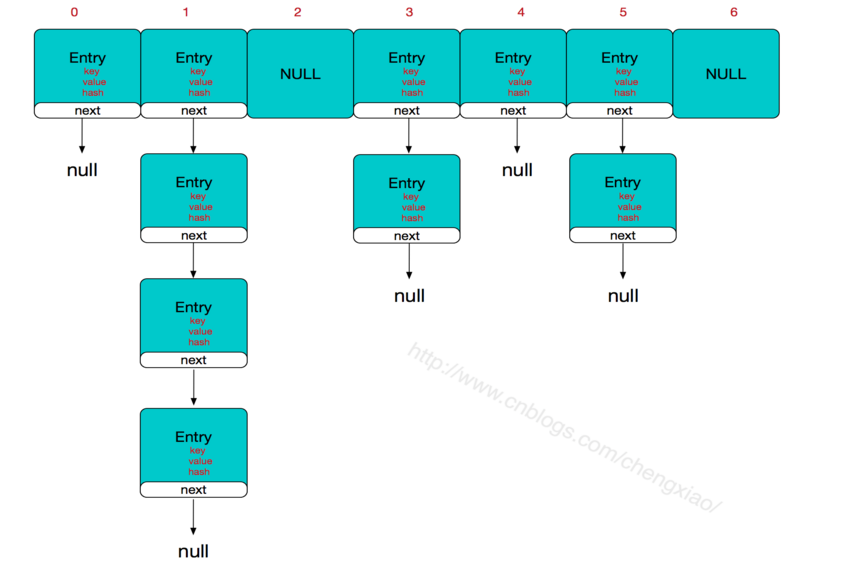HashMap中一些重要的字段

 int threshold;             // 所能容纳的key-value对极限
final float loadFactor;    // 负载因子
int modCount;
int size;

Node[] table的初始化长度length(默认值是16)，Load factor为负载因子(默认值是0.75)，threshold是HashMap所能容纳的最大数据量的Node(键值对)个数。threshold = length * Load factor。也就是说，在数组定义好长度之后，负载因子越大，所能容纳的键值对个数越多。

size这个字段其实很好理解，就是HashMap中实际存在的键值对数量，而modCount字段主要用来记录HashMap内部结构发生变化的次数

 static final int hash(Object key) {
int h;
// h = key.hashCode() 为第一步 取hashCode值
// h ^ (h >>> 16)  为第二步 高位参与运算
return (key == null) ? 0 : (h = key.hashCode()) ^ (h >>> 16);
}

//jdk1.7的源码，jdk1.8没有这个方法，jdk1.8直接使用这个算式而没有封装这个方法，但原理都一样
static int indexFor(int h, int length) {
return h & (length-1);  //第三步 取模运算
}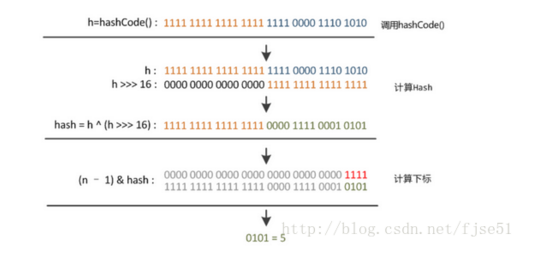HashMap的put方法实现

思路：
1.对key的hashCode()做hash，然后再计算index;
2.如果没冲突直接放到数组里；
3.如果冲突了，以链表的形式存在当前存在元素后；
4.如果冲突导致链表过长(大于等于TREEIFY_THRESHOLD，默认为8)，就把链表转换成红黑树；
5.如果节点已经存在就替换old value(保证key的唯一性)
6.如果数组满了(超过load factor*current capacity)，就要resize扩容。

    public V put(K key, V value) {
return putVal(hash(key), key, value, false, true);
}

/**
*生成hash的方法
*/
static final int hash(Object key) {
int h;
return (key == null) ? 0 : (h = key.hashCode()) ^ (h >>> 16);
}

final V putVal(int hash, K key, V value, boolean onlyIfAbsent,boolean evict) {
Node<K,V>[] tab; Node<K,V> p; int n, i;
//判断table是否为空，
if ((tab = table) == null || (n = tab.length) == 0)
n = (tab = resize()).length;//创建一个新的table数组，并且获取该数组的长度
//根据键值key计算hash值得到插入的数组索引i，如果table[i]==null，直接新建节点添加
if ((p = tab[i = (n - 1) & hash]) == null)
tab[i] = newNode(hash, key, value, null);
else {//如果对应的节点存在
Node<K,V> e; K k;
//判断table[i]的首个元素是否和key一样，如果相同直接覆盖value
if (p.hash == hash &&
((k = p.key) == key || (key != null && key.equals(k))))
e = p;
//判断table[i] 是否为treeNode，即table[i] 是否是红黑树，如果是红黑树，则直接在树中插入键值对
else if (p instanceof TreeNode)
e = ((TreeNode<K,V>)p).putTreeVal(this, tab, hash, key, value);
// 该链为链表
else {
//遍历table[i]，判断链表长度是否大于TREEIFY_THRESHOLD(默认值为8)，大于8的话把链表转换为红黑树，在红黑树中执行插入操作，否则进行链表的插入操作；遍历过程中若发现key已经存在直接覆盖value即可；
for (int binCount = 0; ; ++binCount) {
if ((e = p.next) == null) {
p.next = newNode(hash, key, value, null);
if (binCount >= TREEIFY_THRESHOLD - 1) // -1 for 1st
treeifyBin(tab, hash);
break;
}
if (e.hash == hash &&
((k = e.key) == key || (key != null && key.equals(k))))
break;
p = e;
}
}
// 写入
if (e != null) { // existing mapping for key
V oldValue = e.value;
if (!onlyIfAbsent || oldValue == null)
e.value = value;
afterNodeAccess(e);
return oldValue;
}
}
++modCount;
// 插入成功后，判断实际存在的键值对数量size是否超多了最大容量threshold，如果超过，进行扩容
if (++size > threshold)
resize();
afterNodeInsertion(evict);
return null;
}

HashMap的get方法实现

思路
1.如果是数组里的第一个节点，直接命中；
2.如果有冲突，则通过key.equals(k)去查找对应的entry
若为树，则在树中通过key.equals(k)查找，O(logn)；
若为链表，则在链表中通过key.equals(k)查找，O(n)

public V get(Object key) {
Node<K,V> e;
return (e = getNode(hash(key), key)) == null ? null : e.value;
}

final Node<K,V> getNode(int hash, Object key) {
Node<K,V>[] tab; Node<K,V> first, e; int n; K k;
if ((tab = table) != null && (n = tab.length) > 0 &&
(first = tab[(n - 1) & hash]) != null) {
// 直接命中
if (first.hash == hash && // 每次都是校验第一个node
((k = first.key) == key || (key != null && key.equals(k))))
return first;
// 未命中
if ((e = first.next) != null) {
// 在树中获取
if (first instanceof TreeNode)
return ((TreeNode<K,V>)first).getTreeNode(hash, key);
// 在链表中获取
do {
if (e.hash == hash &&
((k = e.key) == key || (key != null && key.equals(k))))
return e;
} while ((e = e.next) != null);
}
}
return null;
}

HashMap的扩容：由于JDK1.8引入了红黑树，所以我们先使用JDK1.7的代码帮我们理解一下

void resize(int newCapacity) {   //传入新的容量
Entry[] oldTable = table;    //引用扩容前的Entry数组
int oldCapacity = oldTable.length;     //获取旧数组的长度
if (oldCapacity == MAXIMUM_CAPACITY) {  //扩容前的数组大小如果已经达到最大(2^30)了
threshold = Integer.MAX_VALUE; //修改阈值为int的最大值(2^31-1)，这样以后就不会扩容了
return;
}

Entry[] newTable = new Entry[newCapacity];  //初始化一个新的Entry数组
transfer(newTable);                         //！！将数据转移到新的Entry数组里
table = newTable;                           //HashMap的table属性引用新的Entry数组
threshold = (int)(newCapacity * loadFactor);//修改阈值
}

transfer()方法将原有Entry数组的元素拷贝到新的Entry数组里。

 void transfer(Entry[] newTable) {
Entry[] src = table;                   //src引用了旧的Entry数组
int newCapacity = newTable.length;    //获取新数组的长度
for (int j = 0; j < src.length; j++) { //遍历旧的Entry数组
Entry<K,V> e = src[j];             //取得旧Entry数组的每个元素
if (e != null) {
src[j] = null;//释放旧Entry数组的对象引用（for循环后，旧的Entry数组不再引用任何对象）
do {
Entry<K,V> next = e.next;
int i = indexFor(e.hash, newCapacity); //！！重新计算每个元素在数组中的位置
e.next = newTable[i]; //标记
newTable[i] = e;      //将元素放在数组上
e = next;             //访问下一个Entry链上的元素
} while (e != null);
}
}
}

newTable[i]的引用赋给了e.next，也就是使用了单链表的头插入方式，同一位置上新元素总会被放在链表的头部位置；这样先放在一个索引上的元素终会被放到Entry链的尾部(如果发生了hash冲突的话。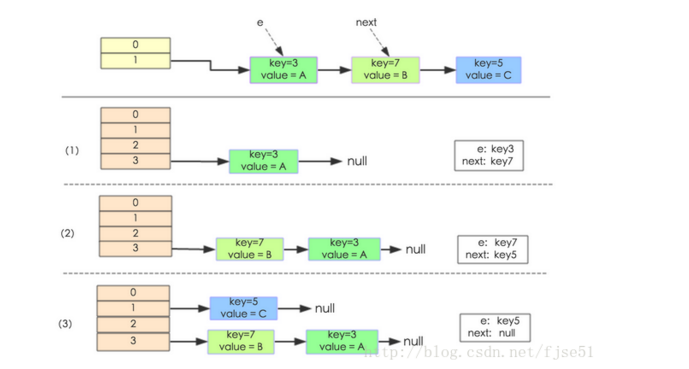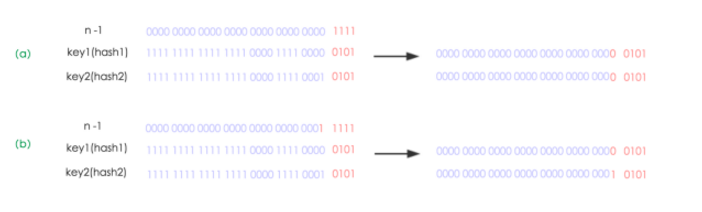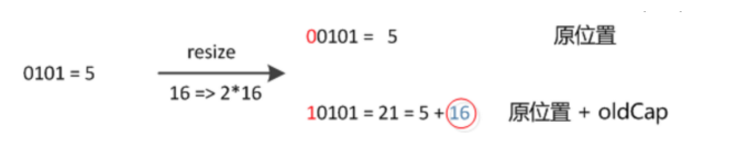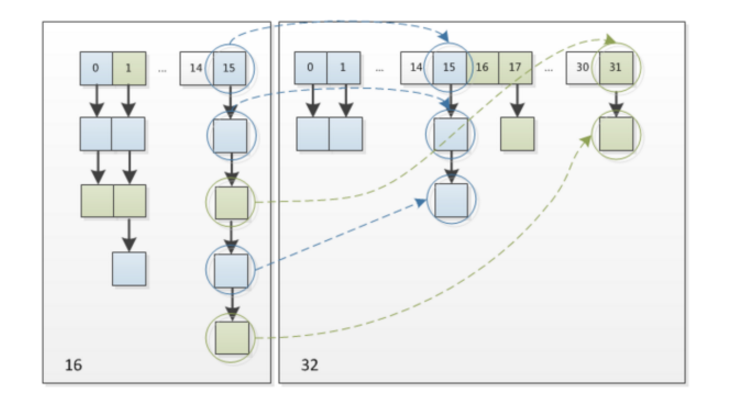1  final Node<K,V>[] resize() {
2     Node<K,V>[] oldTab = table;
3     int oldCap = (oldTab == null) ? 0 : oldTab.length;
4     int oldThr = threshold;
5     int newCap, newThr = 0;
6     if (oldCap > 0) {
7         // 超过最大值就不再扩充了，就只好随你碰撞去吧
8         if (oldCap >= MAXIMUM_CAPACITY) {
9             threshold = Integer.MAX_VALUE;
10             return oldTab;
11         }
12         // 没超过最大值，就扩充为原来的2倍
13         else if ((newCap = oldCap << 1) < MAXIMUM_CAPACITY &&
14                  oldCap >= DEFAULT_INITIAL_CAPACITY)
15             newThr = oldThr << 1; // double threshold
16     }
17     else if (oldThr > 0) // initial capacity was placed in threshold
18         newCap = oldThr;
19     else {               // zero initial threshold signifies using defaults
20         newCap = DEFAULT_INITIAL_CAPACITY;
21         newThr = (int)(DEFAULT_LOAD_FACTOR * DEFAULT_INITIAL_CAPACITY);
22     }
23     // 计算新的resize上限
24     if (newThr == 0) {
25
26         float ft = (float)newCap * loadFactor;
27         newThr = (newCap < MAXIMUM_CAPACITY && ft < (float)MAXIMUM_CAPACITY ?
28                   (int)ft : Integer.MAX_VALUE);
29     }
30     threshold = newThr;
31     @SuppressWarnings({"rawtypes"，"unchecked"})
32         Node<K,V>[] newTab = (Node<K,V>[])new Node[newCap];
33     table = newTab;
34     if (oldTab != null) {
35         // 把每个bucket都移动到新的buckets中
36         for (int j = 0; j < oldCap; ++j) {
37             Node<K,V> e;
38             if ((e = oldTab[j]) != null) {
39                 oldTab[j] = null;
40                 if (e.next == null)
41                     newTab[e.hash & (newCap - 1)] = e;
42                 else if (e instanceof TreeNode)
43                     ((TreeNode<K,V>)e).split(this, newTab, j, oldCap);
44                 else { // 链表优化重hash的代码块
45                     Node<K,V> loHead = null, loTail = null;
46                     Node<K,V> hiHead = null, hiTail = null;
47                     Node<K,V> next;
48                     do {
49                         next = e.next;
50                         // 原索引
51                         if ((e.hash & oldCap) == 0) {
52                             if (loTail == null)
53                                 loHead = e;
54                             else
55                                 loTail.next = e;
56                             loTail = e;
57                         }
58                         // 原索引+oldCap
59                         else {
60                             if (hiTail == null)
61                                 hiHead = e;
62                             else
63                                 hiTail.next = e;
64                             hiTail = e;
65                         }
66                     } while ((e = next) != null);
67                     // 原索引放到bucket里
68                     if (loTail != null) {
69                         loTail.next = null;
70                         newTab[j] = loHead;
71                     }
72                     // 原索引+oldCap放到bucket里
73                     if (hiTail != null) {
74                         hiTail.next = null;
75                         newTab[j + oldCap] = hiHead;
76                     }
77                 }
78             }
79         }
80     }
81     return newTab;
82 }

## 自己实现简单HashMap

//先创建一个键值对类
public class Entry<K, V> {
K key;
V value;

public Entry(K key,V value) {
this.key=key;
this.value=value;
}
}
//自己实现简单的HashMap

public class MyHashMap<K, V> {
//由于HashMap内部是数组加链表，我们就直接使用链表数组了
LinkedList[] array=new LinkedList;

//存放，这里我们没有处理null值，HashMap是允许存放null值的，并且null值总是存放在数组的第一个位置
public void put(K key,V value) {
//创建一个新的entry
Entry e=new Entry<K, V>(key, value);
//将键的hashcode然后模上数组的长度得到索引
int index=key.hashCode()%array.length;
//判断数组当前位置是否为空
if(array[index]==null) {
//没有的话就创建一个链表，放入数组的位置
LinkedList linkedList=new LinkedList();
linkedList.add(e);
array[index]=linkedList;
}else {
//如果不为空，判断当前链表中有没有相同的key，有的话覆盖其value
LinkedList linkedList=array[index];
for(int i=0;i<linkedList.size();i++) {
Entry en=(Entry) linkedList.get(i);
if(en.key.equals(key)) {
en.value=value;
}
}
//没有相同的key就添加该元素到链表中
array[index].add(e);
}

}

//获取元素
public V get(K key) {
int index=key.hashCode()%array.length;
//先判断当前位置是否为空，是的话就判断key是否相当，相同返回Value
if(array[index]!=null) {
LinkedList linkedList=array[index];
for(int i=0;i<linkedList.size();i++) {
Entry en=(Entry) linkedList.get(i);
if(en.key.equals(key)) {
return (V) en.value;
}
}
}
return null;
}

}

## hashcode和equals

hashcode()和equals()都是Object类中的两个方法。我们先来看一下源码。

    //Object中的equals比较两个对象地址是否相同
public boolean equals(Object obj) {
return (this == obj);
}
    //根据本地操作系统生成hashcode，就是对象的内存地址
public native int hashCode();

hashcode不相同，equals一定不同；hashcode相同，equals可能相同，也可能不同。

# HashSet内部原理及实现

HashSet内部基于HashMap来实现的，底层采用HashMap来保存元素。Set集合无序并不可重复。HashSet中的元素都存放在HashMap的key上面，而value中的值都是统一的一个private static final Object PRESENT = new Object();。HashSet跟HashMap一样，都是一个存放链表的数组。

//部分源码分析

public class HashSet<E>
extends AbstractSet<E>
implements Set<E>, Cloneable, java.io.Serializable
{
static final long serialVersionUID = -5024744406713321676L;

//内部使用HashMap
private transient HashMap<E,Object> map;

//实例一个HashMap
public HashSet() {
map = new HashMap<>();
}

public HashSet(Collection<? extends E> c) {
map = new HashMap<>(Math.max((int) (c.size()/.75f) + 1, 16));
addAll(c);
}

//获取迭代器
public Iterator<E> iterator() {
return map.keySet().iterator();
}

//调用map的size方法
public int size() {
return map.size();
}

//调用map的isEmpty方法
public boolean isEmpty() {
return map.isEmpty();
}

//调用map的containsKey方法判断是否包含指定的元素
public boolean contains(Object o) {
return map.containsKey(o);
}

//添加元素，将要添加的元素作为键
public boolean add(E e) {
return map.put(e, PRESENT)==null;
}

//删除元素
public boolean remove(Object o) {
return map.remove(o)==PRESENT;
}

//清除元素
public void clear() {
map.clear();
}

}